# When 15.0 mL of a 8.40×10-4 M ammonium fluoride solution is combined with 25.0 mL of a4.22x10-4 M magnesium bromide solution does a precipitate form?(yes or no)For these conditions the Reaction Quotient, Q, is equal to

Question
2 views

When 15.0 mL of a 8.40×10-4 M ammonium fluoride solution is combined with 25.0 mL of a 4.22×10-4 M magnesium bromide solution does a precipitate form? (yes or no)

For these conditions the Reaction Quotient, Q, is equal to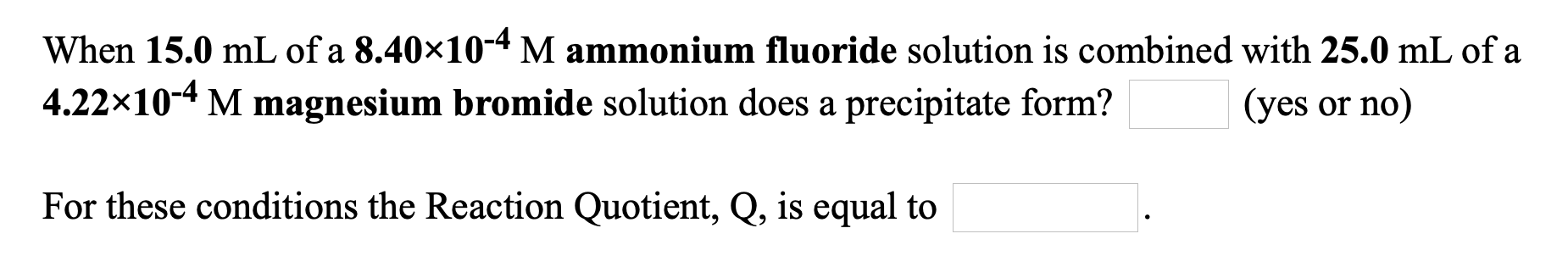help_outlineImage TranscriptioncloseWhen 15.0 mL of a 8.40×10-4 M ammonium fluoride solution is combined with 25.0 mL of a 4.22x10-4 M magnesium bromide solution does a precipitate form? (yes or no) For these conditions the Reaction Quotient, Q, is equal to fullscreen
check_circle

star
star
star
star
star
1 Rating
Introduction:

Precipitate formation during reactions can be governed by the solubility product (Ksp) value of that precipitate. When the product of ions concentration of that compound which is called reaction quotient exceeds value of Ksp , compound starts precipitating out as solid insoluble salt.

Given:

The volume of ammonium fluoride is 15 mL.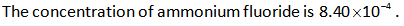The volume of magnesium bromide is 25 mL.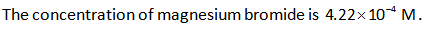Formula and Calculations:

The reaction between ammonium fluoride and magnesium bromide is shown below.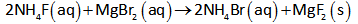In the reaction, 2 moles of ammonium fluoride react with 1 mole of magnesium bromide to form magnesium fluoride and ammonium bromide.

The total volume of solution after the mixing of two compounds is (15+25) =40 mL.

Thus, the concentration of reactants after mixing is calculated as follows,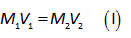Here,

The volume of reactant before mixing is “V1”.

The volume of solution after mixing is “V2”.

The concentration of reactant before mixing is “M1”.

The concentration of reactant after mixing is “M2”.

Substitute the known values for NH4F in equation (I).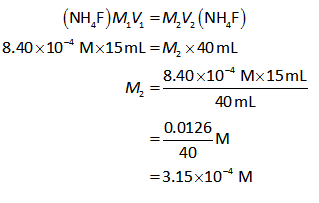Similarly, substitute the known values for MgBr2 in equation (I).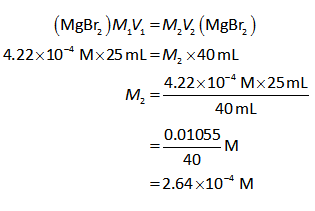Dissociation of magnesium fluoride is shown below.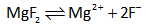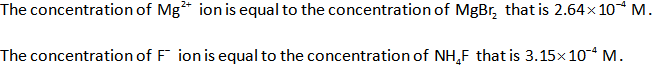Substitute the values in the formula.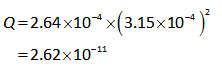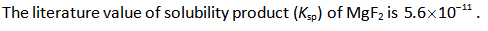As, value of Q less than that of Ksp, precipitation will not occur in the reaction.

...

### Want to see the full answer?

See Solution

#### Want to see this answer and more?

Solutions are written by subject experts who are available 24/7. Questions are typically answered within 1 hour.*

See Solution
*Response times may vary by subject and question.
Tagged in